# Resources tagged with: Graph sketching

Filter by: Content type:
Age range:
Challenge level:

### There are 42 results

Broad Topics > Coordinates, Functions and Graphs > Graph sketching### Polar Flower

##### Age 16 to 18Challenge Level

This polar equation is a quadratic. Plot the graph given by each factor to draw the flower.### Interpolating Polynomials

##### Age 16 to 18Challenge Level

Given a set of points (x,y) with distinct x values, find a polynomial that goes through all of them, then prove some results about the existence and uniqueness of these polynomials.### Maltese Cross

##### Age 16 to 18Challenge Level

Sketch the graph of $xy(x^2 - y^2) = x^2 + y^2$ consisting of four curves and a single point at the origin. Convert to polar form. Describe the symmetries of the graph.### Immersion

##### Age 14 to 16Challenge Level

Various solids are lowered into a beaker of water. How does the water level rise in each case?### Cocked Hat

##### Age 16 to 18Challenge Level

Sketch the graphs for this implicitly defined family of functions.### Power Up

##### Age 16 to 18Challenge Level

Show without recourse to any calculating aid that 7^{1/2} + 7^{1/3} + 7^{1/4} < 7 and 4^{1/2} + 4^{1/3} + 4^{1/4} > 4 . Sketch the graph of f(x) = x^{1/2} + x^{1/3} + x^{1/4} -x### Maths Filler 2

##### Age 14 to 16Challenge Level

Can you draw the height-time chart as this complicated vessel fills with water?### How Many Solutions?

##### Age 16 to 18Challenge Level

Find all the solutions to the this equation.### Pitchfork

##### Age 16 to 18Challenge Level

Plot the graph of x^y = y^x in the first quadrant and explain its properties.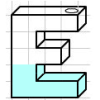### Mathsjam Jars

##### Age 14 to 16Challenge Level

Imagine different shaped vessels being filled. Can you work out what the graphs of the water level should look like?### Exploring Cubic Functions

##### Age 14 to 18Challenge Level

Quadratic graphs are very familiar, but what patterns can you explore with cubics?### How Does Your Function Grow?

##### Age 16 to 18Challenge Level

Compares the size of functions f(n) for large values of n.### Squareness

##### Age 16 to 18Challenge Level

The family of graphs of x^n + y^n =1 (for even n) includes the circle. Why do the graphs look more and more square as n increases?### Curve Hunter

##### Age 14 to 18Challenge Level

This problem challenges you to sketch curves with different properties.### Guessing the Graph

##### Age 14 to 16Challenge Level

Can you suggest a curve to fit some experimental data? Can you work out where the data might have come from?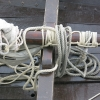### Tangled Trig Graphs

##### Age 16 to 18Challenge Level

Can you work out the equations of the trig graphs I used to make my pattern?### Bird-brained

##### Age 16 to 18Challenge Level

How many eggs should a bird lay to maximise the number of chicks that will hatch? An introduction to optimisation.### Guess the Function

##### Age 16 to 18Challenge Level

This task depends on learners sharing reasoning, listening to opinions, reflecting and pulling ideas together.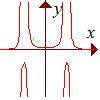### Rational Request

##### Age 16 to 18Challenge Level

Can you make a curve to match my friend's requirements?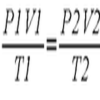### Ideal Axes

##### Age 16 to 18Challenge Level

Explore how can changing the axes for a plot of an equation can lead to different shaped graphs emerging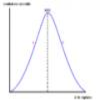### Graphic Biology

##### Age 16 to 18Challenge Level

Several graphs of the sort occurring commonly in biology are given. How many processes can you map to each graph?### What's That Graph?

##### Age 14 to 18Challenge Level

Can you work out which processes are represented by the graphs?### A Close Match

##### Age 16 to 18Challenge Level

Can you massage the parameters of these curves to make them match as closely as possible?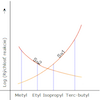### Reaction Types

##### Age 16 to 18Challenge Level

Explore the rates of growth of the sorts of simple polynomials often used in mathematical modelling.### Whose Line Graph Is it Anyway?

##### Age 16 to 18Challenge Level

Which line graph, equations and physical processes go together?### Quartics

##### Age 16 to 18Challenge Level

Investigate the graphs of y = [1 + (x - t)^2][1 + (x + t^)2] as the parameter t varies.### Back Fitter

##### Age 14 to 18Challenge Level

10 graphs of experimental data are given. Can you use a spreadsheet to find algebraic graphs which match them closely, and thus discover the formulae most likely to govern the underlying processes?### Area L

##### Age 16 to 18Challenge Level

By sketching a graph of a continuous increasing function, can you prove a useful result about integrals?### Scientific Curves

##### Age 16 to 18Challenge Level

Can you sketch these difficult curves, which have uses in mathematical modelling?### Curve Match

##### Age 16 to 18Challenge Level

Which curve is which, and how would you plan a route to pass between them?### Parabolic Patterns

##### Age 14 to 18Challenge Level

The illustration shows the graphs of fifteen functions. Two of them have equations y=x^2 and y=-(x-4)^2. Find the equations of all the other graphs.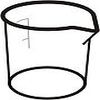### Brimful

##### Age 16 to 18Challenge Level

Can you find the volumes of the mathematical vessels?### Curve Fitter

##### Age 14 to 18Challenge Level

This problem challenges you to find cubic equations which satisfy different conditions.### Bio Graphs

##### Age 14 to 16Challenge Level

What biological growth processes can you fit to these graphs?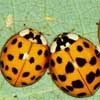### Spot the Difference

##### Age 16 to 18 ShortChallenge Level

If you plot these graphs they may look the same, but are they?### Slide

##### Age 16 to 18Challenge Level

This function involves absolute values. To find the slope on the slide use different equations to define the function in different parts of its domain.### Equation Matcher

##### Age 16 to 18Challenge Level

Can you match these equations to these graphs?### Folium of Descartes

##### Age 16 to 18Challenge Level

Investigate the family of graphs given by the equation x^3+y^3=3axy for different values of the constant a.### Sine Problem

##### Age 16 to 18Challenge Level

In this 'mesh' of sine graphs, one of the graphs is the graph of the sine function. Find the equations of the other graphs to reproduce the pattern.### More Parabolic Patterns

##### Age 14 to 18Challenge Level

The illustration shows the graphs of twelve functions. Three of them have equations y=x^2, x=y^2 and x=-y^2+2. Find the equations of all the other graphs.### Witch of Agnesi

##### Age 16 to 18Challenge Level

Sketch the members of the family of graphs given by y = a^3/(x^2+a^2) for a=1, 2 and 3.### Integration Matcher

##### Age 16 to 18Challenge Level

Can you match the charts of these functions to the charts of their integrals?